Select Page

# Example of Elimination Of Epsilon (ε)

As we already see the Conversion of Epsilon-NFA to NFA. Let explain the example of elimination of epsilon from NFA.

## Example

Consider the following epsilon NFA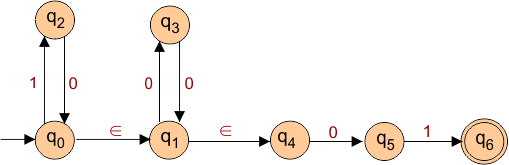### Solution

In the above epsilon NFA,  there are two epsilon exist. First we remove the epsilon which is for away from intial state. So, first we remove the epsilon which exist in q1 and q4.

suppose q1 is V1 and q4 is V2 because epsilon exist between these two states. Now apply the steps of epsilon removal.

### Step 01: Find all edges starting from V2

• The only input “0” is starting from V2 (q4) and going to q5.

### Step 02: Remove the epsilon. All Finding edges from V2 are Duplicated to V1 without changing edge labels.

As the Input “0” is going to q5 from V2 (q2). So duplicate will goes from V1 (q1) to q5. So, duplicated edge with their lables are given below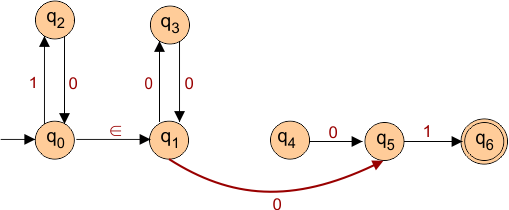### Step 03: if V1 is initial state, make V2 initial State

As V1 (q1) is not a initial state. So V2(q2) will be unchanged and NFA  of Step 2 remain Same.

### Step 04: if V2 is final state, make V1 final State

As V2 (q4) is not a final state. So, V1(q1) will unchanged and NFA  of Step 2 remain Same.

### Step 05: Remove all dead states

A state which is unreachable is called dead state. In above NFA, q4 is dead state. So, NFA after removal of first epsilon and dead state is given below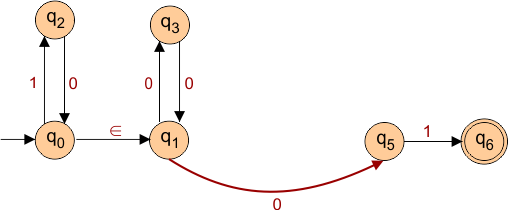### Now Repeat the Above 5 steps again for elimination of 2nd epsilon.

After first 5 steps epsilon- NFA , epsilon exist between q0 and q1. Again suppose q0 is V1 and q2 is V2.

### Step 01: Find all edges starting from V2

• The only input “0” is starting from V2 (q2) and going to q3.

### Step 02: Remove the epsilon. All Finding edges from V2 are Duplicated to V1 without changing edge labels.

As the Input “0” is going to q3 from V2 (q1). So duplicate will goes from V1 (q0) to q3. So, duplicated edge with their labels are given below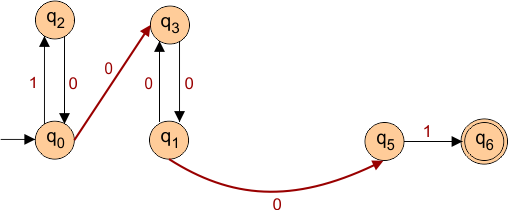### Step 03: if V1 is initial state, make V2 initial State

As V1 (q0) is a initial state. So V2(q1) will be change to final . As given below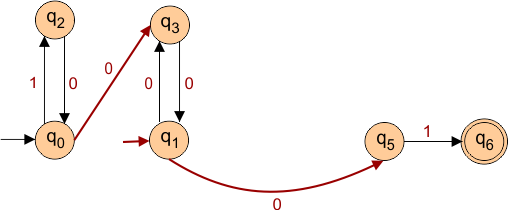### Step 04: if V2 is final state, make V1 final State

As V2 (q1) is not a final state. So, V1(q1) will unchanged and NFA of above step3 will remain the same.

### Step 05: Remove all dead states

No dead state found. The above NFA of step 3 will remain the same. So, the final NFA after elimination of both epsilons and dead state is given belowHelp Other’s By Sharing…